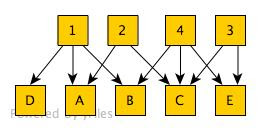## Tuesday, September 11, 2007

### Matching names in phylogeny data files

In an earlier post I described the TBMap database (doi:10.1186/1471-2105-8-158), which contains a mapping of TreeBASE taxon names onto names in other databases. While this is one step towards making it easier to query TreeBASE, what I'd really like is to link the data in TreeBASE to sources such as GenBank and specimen databases. Part of the challenge of doing this (and also doing it more generally, such as taking a NEXUS file from the web and using that) is that the names people use in a NEXUS data file are often not the actual taxonomic names. So, if I take a table from a paper that lists GenBank accession numbers, voucher specimens, etc., I'm left with the problem of matching two sets of names -- those in the data file to those in the table.

For example, this is a TreeBASE taxon Apomys_sp._B_145699. Using a script I grabbed the sequences in this study and constructed a table listing the name and specimen voucher for each sequence. The name corresponding to the TreeBASE taxon is Apomys "sibuyan b" FMNH 145699. Clearly a similar string, but not the same.

The approach I've taken is to compare strings using the longest common subsequence algorithm. Below are the two strings, with their longest common subsequence highlighted in green.

Apomys
_sp._B_145699

Apomys "sibuyan b" FMNH 145699

The length of this subsequence is used to compute a measure of distance between the two strings. If len1 is the length of the first string, len2 is the length of the second string, and lcs is the length of the longest common subsequence, then

d = (len1 + len2) - 2 × lcs

We can normalise this by dividing by len1 + len2, so that d ranges from 0 (identical) to 1.0 (no similarity).

So, now we have a measure of how similar the strings are, I still need to find the set of matchings between file and table names. This can be modelled an example of a maximum weight bipartite matching problem. Given a bipartite graph, we want to find the matching with the highest weight. A "matching" is where each node is connected to just one other node. For example, given this graph:a maximum weight matching is:In this example, four pairs of nodes are matched, one is "orphaned". Applying this to the data matching problem, I take a list of names form the NEXUS file, and a list of names from the paper (or supplementary data file, etc.) and compute a maximum weight matching. Because I'm looking for the maximum weighted matching I actually want similarity of names, hence I subtract the normalised d from 1.0.

So, the algorithm consists of taking the two lists of names (taxa from dataset and names from a table), computing the distance between all pairs of names, then obtain the maximum weight bipartite matching. Because for large sets of names the n × m the bipartite graph becomes huge, and because in practice most matches are poor, for each node in the graph I only draw the edges corresponding to the five most similar pairs of strings.